# ...find the design angle of attack of my wing?

The lift of a wing of finite span at a certain angle of attack is less than the lift of a two dimensional airfoil (which corresponds to a wing of infinite span). In general, the lift loss due to the wing tips is more pronounced, as the wing span is reduced.

This effect also means that the angle of attack required to reach the same lift must be higher as the wing span is getting smaller. The relation mainly depends on the aspect ratio of the wing and can be approximated as follows.

Procedure (abbreviations and symbols are listed at the end of this page):

• Calculate the aspect ratio of your wing (AR):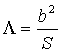• Find the slope a0 of the 2D airfoil lift curve from a calculated or experimental polar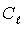versus angle of attack a. Convert from [1/°] to [1/radian] by multiplying with 180° and dividing by p. The slope can be calculated by taking the lift coefficients at two angles of attack and subtracting them to give the DCl the same must be done with the angles to yield Da.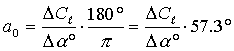(In general this value will be in the order of 5-6)

• Transform the slope of the 2D lift curve to the finite wing. The formula depends on the aspect ratio AR:
 Case AR <= 4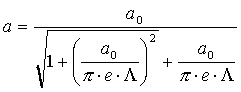Case AR > 4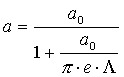Finally:

• either calculate the lift coefficient CL for a given angle of attack for the finite wing: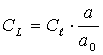• or calculate the angle of attack of the wing (alfa3D) required to reach the same lift coefficient as the two dimensional airfoil at (alfa2D):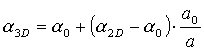Symbol Description
b wing span
e span efficiency factor, typically between 0.85 and 0.95
S wing area
a0 lift slope dCL/dalfa of the infinite wing (2D airfoil)
a lift slope dCL/dalfa of the finite wing (3D wing)
a0 2D zero lift angle of the infinite wing (2D airfoil)
a2D 2D angle of attack of the infinite wing (2D airfoil)
a3D 3D angle of attack of the finite wing (3D wing)lift coefficient of the infinite wing (2D wing)
CL lift coefficient of the finite wing (3D wing)Due to the increasing amount of SPAM mail, I have to change this e-Mail address regularly. You will always find the latest version in the footer of all my pages.

It might take some time until you receive an answer and in some cases you may even receive no answer at all. I apologize for this, but my spare time is limited. If you have not lost patience, you might want to send me a copy of your e-mail after a month or so.
This is a privately owned, non-profit page of purely educational purpose. Any statements may be incorrect and unsuitable for practical usage. I cannot take any responsibility for actions you perform based on data, assumptions, calculations etc. taken from this web page.